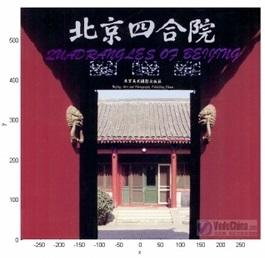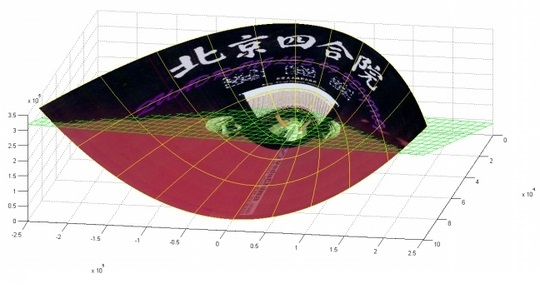# 机器学习：核函数的一个小题目

----------------------------------------------------------------------

（1）核函数

K(x,z) =<Φ(x),Φ(z) > (1)// 即映射// 即核函数----------------------------------------------------------------------

（2）核函数要满足一个必备条件——Mercer's Condition

Mercer定理：任何半正定的函数都可以作为核函数。所谓半正定的函数f(xi,xj)，是指拥有训练数据集合（x1,x2,...xn)，我们定义一个矩阵的元素aij = f(xi,xj)，这个矩阵式n*n的，如果这个矩阵是半正定的，那么f(xi,xj)就称为半正定的函数。This is analogous to the idea of a positive-semidefinite matrix, which is a matrix M satisfying z^{T}Mz\geq0. The quantity z^{T}Mz can be thought of as a double sum, whereas the above integral is also analogous to a double sum.$K(x, y)=1\,$$\iint g(x)g(y)\,dx dy = \int\! g(x) \,dx \int\! g(y) \,dy = \left(\int\! g(x) \,dx\right)^2$

----------------------------------------------------------------------

（3）常用的核函数

1) 线性核// 直接求内积

2) 多项式核// 带参数、偏移、指数的内积

3) 径向基核// 高斯核为典型（带参数）

4) Sigmoid核// 多项式核求双曲正切

1）线性核函数K(x,xi) =x·xi；
2）多项式核函数K(x,xi)=(x·xi+1)^d, d=1,2,…,N；
3）高斯核函数K(x,xi) =exp(-||x-xi||2/2σ2；
4）感知器核函数K(x,xi) =tanh(βxi+b)；
5）样条核函数K(x,xi) = B2n+1(x-xi)。

----------------------------------------------------------------------

（4）开头给出三角形问题的个人思路©️2019 CSDN 皮肤主题: 编程工作室 设计师: CSDN官方博客